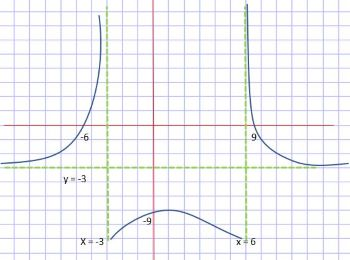# Find the possible formula for the function in the figure below. (Graph)

## Question:

Find the possible formula for the function in the figure below.## Rational Functions

If a function is the ratio of two polynomials, it's called a rational function. This function may have vertical asymptotes when the denominator is zero, and it may have a horizontal asymptote depending on the degree of the numerator and denominator.

The graph given has two vertical asymptotes, at {eq}x = -3 {/eq} and at {eq}x = 6 {/eq}, and a horizontal asymptote, at {eq}y = -3 {/eq}. Thus, we...

Become a Study.com member to unlock this answer! Create your account